# Test: Sets- 1

## 30 Questions MCQ Test RRB JE for Computer Science Engineering | Test: Sets- 1

Description
Attempt Test: Sets- 1 | 30 questions in 90 minutes | Mock test for Computer Science Engineering (CSE) preparation | Free important questions MCQ to study RRB JE for Computer Science Engineering for Computer Science Engineering (CSE) Exam | Download free PDF with solutions
QUESTION: 1

### A binary operation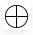on a set of integers is defined as xy = x2 + y2. Which one of the following statements is TRUE about?

Solution:

Associativity: A binary operation ∗ on a set S is said to be associative if it satisfies the associative law: a ∗ (b ∗c) = (a ∗b) ∗c for all a, b, c ∈S.
Commutativity: A binary operation ∗ on a set S is said to be commutative if it satisfies the condition: a ∗b=b ∗a for all a, b, ∈S. In this case, the order in which elements are combined does not matter.
Solution: Here a binary operation on a set of integers is defined as x ⊕ y = x2 + y2. for Commutativity: x ⊕ y= y ⊕ x. LHS=> x ⊕ y= x^2+ y^2 RHS=> y ⊕ x= y^2+x^2 LHS = RHS. hence commutative. for Associativity: x ⊕ (y ⊕ z) =(x ⊕ y) ⊕ z LHS=> x ⊕ (y ⊕ z) = x ⊕ ( y^2+z^2)= x^2+(y^2+z^2)^2 RHS=> (x ⊕ y) ⊕ z= ( x^2+y^2) ⊕ z=(x^2+y^2)^2+z^2 So, LHS ≠ RHS, hence not associative.

Another Solution : [Tex]oplus[/Tex] commutative as x[Tex]oplus[/Tex]y is always same as y[Tex]oplus[/Tex]x. [Tex]oplus[/Tex] is not associative as (x[Tex]oplus[/Tex]y)[Tex]oplus[/Tex]z is (x^2 + y^2)^2 + z^2, but x[Tex]oplus[/Tex](y[Tex]oplus[/Tex]z) is x^2 + (y^2 + z^2)^2.

QUESTION: 2

### Consider the set S = {1, ω, ω2}, where ω and w2 are cube roots of unity. If * denotes the multiplication operation, the structure (S, *) forms

Solution:

A group is a set of elements together with an operation that combines any two of its elements to form a third element also in the set while satisfying four conditions called the group axioms, namely closure, associativity, identity and invertibility.

QUESTION: 3

### Which one of the following in NOT necessarily a property of a Group?

Solution:

A group is a set, G, together with an operation • (called the group law of G) that combines any two elements a and b to form another element, denoted a • b or ab. To qualify as a group, the set and operation, (G, •), must satisfy four requirements known as the group axioms: Closure For all a, b in G, the result of the operation, a • b, is also in G.b Associativity For all a, b and c in G, (a • b) • c = a • (b • c). Identity element There exists an element e in G, such that for every element a in G, the equation e • a = a • e = a holds. Such an element is unique (see below), and thus one speaks of the identity element. Inverse element For each a in G, there exists an element b in G such that a • b = b • a = e, where e is the identity element. The result of an operation may depend on the order of the operands. In other words, the result of combining element a with element b need not yield the same result as combining element b with element a; the equation a • b = b • a may not always be true. This equation always holds in the group of integers under addition, because a + b = b + a for any two integers (commutativity of addition). Groups for which the commutativity equation a • b = b • a always holds are called abelian groups (in honor of Niels Abel)

QUESTION: 4

Consider the binary relation R = {(x, y), (x, z), (z, x), (z, y)} on the set {x, y, z}. Which one of the following is TRUE?

Solution:

R is not symmetric as (x, y) is present, but (y, x) is not present in R. R is also not antisymmetric as both (x, z) and (z, x) are present in R

QUESTION: 5

For the composition table of a cyclic group shown below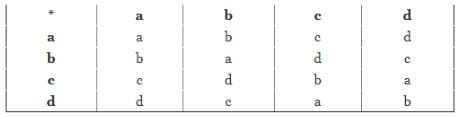Q.
Which one of the following choices is correct?

Solution:

Check for all:- a1 = a ,
a2 = a * a = a
a3 = a2 * a = a * a = a
a is not the generator since we are not able to express other members of the group in powers of a
Check for c -
c1 = c
c2 = c * c = b
c3 = c2 * c = b * c = d
c4 = c2 * c2 = b * b = a

We are able to generate all the members of the group from c , Hence c is the generator
Similarly check for d

QUESTION: 6

If P, Q, R are subsets of the universal set U, then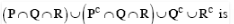Solution: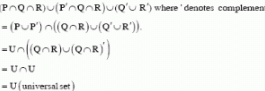QUESTION: 7

Let S be a set of nelements. The number of ordered pairs in the largest and the smallest equivalence relations on S are:

Solution:

Consider an example set, S = (1,2,3)

Equivalence property follows, reflexive, symmetric and transitive

Largest ordered set are s x s = {(1,1) (1,2) (1,3) (2,1) (2,2) (2,3) (3,1) (3,2) (3,3)} which are 9 which equal to 3^2 = n^2

Smallest ordered set are {(1,1) (2,2) (3,3)} which are 3 and equals to n. number of elements.

QUESTION: 8

How many different non-isomorphic Abelian groups of order 4 are there

Solution:

2 can be written as 2 power 2.
Number of partitioning of 2 = no. of non isomorphic abelian groups
2 can be partitioned as {(2),(1,1)}

QUESTION: 9

Consider the set S = {a,b,c,d}. consider the following 4 partitions π1, π2, π3, π4 on S : π1 =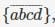π2 =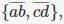π3 =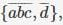π4 =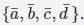Let ρ be the partial order on the set of partitions S' = {π123, π4} defined as follows : π ρ πj if and only if πi refines πj . The poset diagram for (S', ρ) is :

Solution:
QUESTION: 10

Consider the set of (column) vector defined by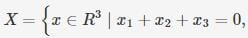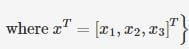Which of the following is True ?

Solution:

To be basis of subspace x, 2 conditions are to be fulfilled 1) They must span x2) The vectors have to be linearly independent 1)the general solution of x1+x2+x3=0 is [-x2-x3 , x2 , x3]^T (Transpose) Which gives two linearly independent solutions by by assuming x2 = 1 and x3 = 0 and next x3 = 1 and x2 = 0 gives [-1,1,0]^T and [-1,0,1]^T respectively. Since both of these can be generated by linear combinations of [1,-1,0]^T & [-1,0,1]^T given in question, it span x. 2) Above set of column vector is linearly independent because one cannot be obtained from another by scalar multiplication (second method rank is 2..that is why linearly independent)

QUESTION: 11

Let X, Y, Z be sets of sizes x, y and z respectively. Let W = X x Y. Let E be the set of all subsets of W. The number of functions from Z to E is:

Solution:

Number of functions from a set A of size m to set B of size n is nm, because each of the m elements of A has n choices for mapping. Now here m=|Z|=z, and n=|E|=2xy because number of subsets of a set of size n is 2n, and here set W has size of xy.
So number of functions from Z to E = (2xy)z=2xyz. So option (D) is correct.

QUESTION: 12

The set {1, 2, 3, 5, 7, 8, 9} under multiplication modulo 10 is not a group. Given below are four plausible reasons. Which one of them is false?

Solution:

A is not closed under multiplication as we may get 0 after multiplication and 0 is not present in set. 2 doesn't have an inverse as there is no x such that (2*x) mod 10 is 1. 3 has an inverse as (3*7) mod 10 is 1. 8 doesn't have an inverse as there is no x such that (2*x) mod 10 is 1.

QUESTION: 13

A relation R is defined on ordered pairs of integers as follows: (x,y) R(u,v) if x < u and y > v. Then R is: Then R is:

Solution:

A relation R is defined on ordered pairs of integers as follows: (x,y) R(u,v) if x < u and y > v. Then R is: Then R is: (A) Neither a Partial Order nor an Equivalence Relation (B) A Partial Order but not a Total Order (C) A Total Order (D) An Equivalence Relation

Solution:

An equivalence relation on a set x is a subset of x*x, i.e., a collection R of ordered pairs of elements of x, satisfying certain properties. Write “x R y" to mean (x,y) is an element of R, and we say "x is related to y," then the properties are 1. Reflexive: a R a for all a Є R, 2. Symmetric: a R b implies that b R a for all a,b Є R 3. Transitive: a R b and b R c imply a R c for all a,b,c Є R.

An partial order relation on a set x is a subset of x*x, i.e., a collection R of ordered pairs of elements of x, satisfying certain properties. Write “x R y" to mean (x,y) is an element of R, and we say "x is related to y," then the properties are

1. Reflexive: a R a for all a Є R, 2. Anti-Symmetric: a R b and b R a implies that for all a,b Є R 3. Transitive: a R b and b R c imply a R c for all a,b,c Є R.

An total order relation a set x is a subset of x*x, i.e., a collection R of ordered pairs of elements of x, satisfying certain properties. Write “x R y" to mean (x,y) is an element of R, and we say "x is related to y," then the properties are

1. Reflexive: a R a for all a Є R, 2. Symmetric: a R b implies that b R a for all a,b Є R 3. Transitive: a R b and b R c imply a R c for all a,b,c Є R. 4. Comparability : either a R b or b R a for all a,b Є R.

As given in question, a relation R is defined on ordered pairs of integers as follows: (x,y) R(u,v) if x < u and y > v , reflexive property is not satisfied here , because there is > or < relationship between (x ,y) pair set and (u,v) pair set . Other way , if there would have been x <= u and y>= v (or x=u and y=v) kind of relation amongs elements of sets then reflexive property could have been satisfied. Since reflexive property in not satisfied here , so given realtion can not be equivalence ,partial order or total order relation.So ,Answer (A) is true .

QUESTION: 14

Let S denote the set of all functions f: {0,1}4 -> {0,1}. Denote by N the number of functions from S to the set {0,1}.

Q.
The value of Log2Log2N is ______.

Solution:

The given mapping S is defined by f:{0,1}^4 -> {0,1}.
So, number of functions from S will be 2^16.
Now N is defined by f : S-> {0,1}.
So Number of functions from S to {0,1} will be 2^S.
Hence log2log2N = log2S = 16

QUESTION: 15

Consider the following relation on subsets of the set S of integers between 1 and 2014. For two distinct subsets U and V of S we say U < V if the minimum element in the symmetric difference of the two sets is in U. Consider the following two statements:

S1: There is a subset of S that is larger than every other subset.
S2: There is a subset of S that is smaller than every other subset.

Q. Which one of the following is CORRECT?

Solution:

According to the given information :
S1 is true because NULL set is smaller than every other set.
S2 is true because the UNIVERSAL set {1, 2, ..., 2014} is larger than every other set.

Thus, both S1 and S2 are true.

Please comment below if you find anything wrong in the above post.

QUESTION: 16

Let X and Y be finite sets and f: X -> Y be a function. Which one of the following statements is TRUE?

Solution:

Let x = {a, b, c} and y = {1, 2} A Function f maps each element of x to 1 in y. f(a)=1 , f(b)=1 , f(c) =1 A = {a, b} B = {b, c}
----------------------------------------------
A] |f(A u B) | = |f({a, b, c})| = 3 | f(A)|+|f(B)| = 2 + 2 = 4 , LHS != RHS.
----------------------------------------------
B] f(A ∩ B) = f({b}) = { 1 } f(A) ∩ f(B) = {1, 1} ∩ {1, 1} = {1, 1} LHS != RHS
-----------------------------------------------
C] |f(A ∩ B)| = |f({b})| = |{ 1 }| = 1 min{|f(A)|,|f(B)|} = min(2,2) = 2 LHS != RHS
-----------------------------------------------
D] In a function a value can be mapped only to one value.

QUESTION: 17

Let G be a group with 15 elements. Let L be a subgroup of G. It is known that L != G and that the size of L is at least 4.

Q. The size of L is __________.

Solution:

This is according to Lagrange's theorem that states that the order of subgroup should divide the order of group. Subgroups of G can be of order 1,3,5 or 15 and according to the conditions and choices, B is the right answer.

QUESTION: 18

If V1 and V2 are 4-dimensional subspaces of a 6-dimensional vector space V, then the smallest possible dimension of V1 ∩ V2 is ______.

Solution:

First, note that V1+V2 is still in V, so dim(V1+V2)≤ 6. We know that dim(V1+V2)=dimV1+dimV2−dim(V1∩V2).
So 6≥dim(V1+V2)=dimV1+dimV2−dim(V1∩V2) dim(V1∩V2)≥4+4−6=2. The answer is B.

QUESTION: 19

There are two elements x, y in a group (G,∗) such that every element in the group can be written as a product of some number of x's and y's in some order. It is known that

x ∗ x = y ∗ y = x ∗ y ∗ x ∗ y = y ∗ x ∗ y ∗ x = e

Q.
where e is the identity element. The maximum number of elements in such a group is __________.

Solution:

x * x = e, x is its own inverse
y * y = e, y is its own inverse

(x*y) * (x* y) = e, x*y is its own inverse

(y*x) * (y*x) = e, y*x is its own inverse
also x*x*e = e*e can be rewritten as follows

x*y*y*x = e*y*y*e = e, (Since y *y = e)

(x*y) * (y*x) = e shows that (x *y) and (y *x) are each other’s inverse and we already know that (x*y) and (y*x) are inverse of its own.

As per (G,*) to be group any element should have only one inverse element (unique)

This implies x*y = y*x (is one element)
So the elements of such group are 4 which are {x, y, e, x*y}.

See following definition of group from wikipedia. A group is a set, G, together with an operation • (called the group law of G) that combines any two elements a and b to form another element, denoted a • b or ab. To qualify as a group, the set and operation, (G, •), must satisfy four requirements known as the group axioms: Closure For all a, b in G, the result of the operation, a • b, is also in G.b[›] Associativity For all a, b and c in G, (a • b) • c = a • (b • c). Identity element There exists an element e in G, such that for every element a in G, the equation e • a = a • e = a holds. Such an element is unique (see below), and thus one speaks of the identity element. Inverse element For each a in G, there exists an element b in G such that a • b = b • a = e, where e is the identity element.

QUESTION: 20

Consider the set of all functions f: {0,1, … ,2014} → {0,1, … ,2014} such that f(f(i)) = i, for all 0 ≤ i ≤ 2014. Consider the following statements:

P. For each such function it must be the case that for every i, f(i) = i.

Q. For each such function it must be the case that for some i, f(i) = i.

R. Each such function must be onto.

Q.
Which one of the following is CORRECT?

Solution:

This kind of functions are called identity functions. We assume f(i) = k. So, f(k) = i. Now, since the values of ' i ' and ' j ' would be same for atleast some values if the domain and co - domain intersect, which is true for the given question, Q is definitely true. But this might not happen for all the values of ' i ', hence, P is not always true. Now, ' i ' ranges from 0 to 2014, so, it takes 2015 possible values. From the definition of a function, we know that for each input to the function, we have a unique output. Also, in the given question, domain and co - domain are exactly same. Therefore, the function is onto and hence, R is definitely true.   Thus, the correct option is B.

QUESTION: 21

Let E, F and G be finite sets. Let X = (E ∩ F) - (F ∩ G) and Y = (E - (E ∩ G)) - (E - F). Which one of the following is true?

Solution:

If we draw the venn diagrams of both X and Y, we find that both cover exactly same  region (shown in figure below).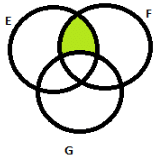So option (C) is correct.

QUESTION: 22

Given a set of elements N = {1, 2, ..., n} and two arbitrary subsets A⊆N and B⊆N, how many of the n! permutations π from N to N satisfy min(π(A)) = min(π(B)), where min(S) is the smallest integer in the set of integers S, and π(S) is the set of integers obtained by applying permutation π to each element of S?

Solution:

First let us understand what question is asking. So π is a function from N to N, which just permutes the elements of N, so there will be n! such permutations. Now given a particular π i.e. given a particular permutation scheme, we have to find number of permutations out of these n! permuations in which minimum elements of A and B after applying π to them are same. So for example, if N = {1,2,3}, π is {2,3,1}, and if A is {1,3}, then π(A) = {2,1}. Now number of elements in A ∪ B is |A ∪ B|. We can choose permutations for A ∪ B in nC|A∪B| ways. Note that here we are just choosing elements for permutation, and not actually permuting. Let this chosen set be P. Now once we have chosen numbers for permutations, we have to select mapping from each element of A ∪ B to some element of P. So first of all, to achieve required condition specified in question, we have to map minimum number in P to any of the number in A ∩ B, so that min(π(A)) = min(π(B)). We can do this in |A∩B| ways, since we can choose any element of |A∩B| to be mapped to minimum number in P. Now we come to permutation. We can permute numbers in P in |A∪B-1|! ways, since one number (minimum) is already fixed. Moreover, we can also permute remaining n - |A∪B-1| in (n - |A∪B-1|)! ways, so total no. of ways = nC|A∪B|∗|A∩B|∗|A∪B−1|!∗(n−|A∪B−1|)!=n!|A∩B||A∪B| So option (C) is correct. Note: Some answer keys on web have shown answer as option (D), which is clearly incorrect. Suppose |A ∪ B| = 3, and |A ∩ B| = 1, and n = 4, then option (D) evaluates to 14=0.25, which doesn't make sense.

QUESTION: 23

Let S = {1,2,3, ....... , m}, m >3. Let X1 ...... Xn be subset of S each of size 3. Define a function f from S to the set of atural numbers as, f(i) is the number of sets X that contain the element i. That is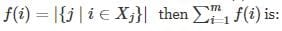Solution:

First of all, number of subsets of S of size 3 is mC3 i.e. n=mC3. Now we count number of subsets in which a particular element i appears, that will be (m−1)C2, because 1 element is already known, and we have to choose 2 elements from remaining m-1 elements. [Tex]sumlimits_{i=1}^{m} f(i) = m * ^{m-1}mathrm{C}_2 = 3 * ^mmathrm{C}_3 = 3n[/Tex]

QUESTION: 24

Let A, B and C be non-empty sets and let X = (A - B) - C and Y = (A - C) - (B - C). Which one of the following is TRUE?

Solution:

We can solve it by making Venn diagram

QUESTION: 25

The following is the Hasse diagram of the poset [{a, b, c, d, e}, ≤]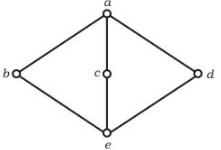The poset is

Solution:

It is a lattice but not a distributive lattice.

Table for Join Operation of above Hesse diagram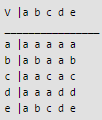Table for Meet Operation of above Hesse diagram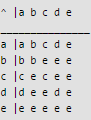Therefore for any two element p, q in the lattice (A,<=) p <= p V q ; p^q <= p This satisfies for all element (a,b,c,d,e).
which has 'a' as unique least upper bound and 'e' as unique greatest lower bound.
The given lattice doesn't obey distributive law, so it is not distributive lattice,

Note that for b,c,d we have distributive law
b^(cVd) = (b^c) V (b^d). From the diagram / tables given above we can verify as follows,
(i) L.H.S. = b ^ (c V d) = b ^ a = b
(ii) R.H.S. = (b^c) V (b^d) = e v e = e
b != e which contradict the distributive law. Hence it is not distributive lattice. so, option (B) is correct.

QUESTION: 26

The set {1, 2, 4, 7, 8, 11, 13, 14} is a group under multiplication modulo 15. The inverses of 4 and 7 are respectively

Solution:

We know that,
For a number 'n', (n) x (n') = I, where
n' = inverse of 'n' I = Identity element Now, the identity element for multiplication is 1. So, we need to find two numbers 'm' and 'n' such that (4 x m) % 15 = 1 and (7 x n) % 15 = 1,
where 'm' is the inverse of 4 and 'n' is the inverse of 7, and both 'm' and 'n' belong to the given set. Thus, from the given set, it can be easily identified by putting values that m = 4 and n = 13. So, C is the correct choice.
Please comment below if you find anything wrong in the above post.

QUESTION: 27

Let R and S be any two equivalence relations on a non-empty set A. Which one of the following statements is TRUE?

Solution:
QUESTION: 28

Let f: B → C and g: A → B be two functions and let h = f o g. Given that h is an onto function. Which one of the following is TRUE?

Solution:

A function f: X → Y is called on-to function if for every value in set Y, there is a value in set X.

Given that, f: B → C and g: A → B and h = f o g.
Note that the sign o represents composition.
h is basically f(g(x)). So h is a function from set A to set C.
It is also given that h is an onto function which means for every value in C there is a value in A.

We map from C to A using B. So for every value in C, there must be a value in B. It means f must be onto. But g may or may not be onto as there may be some values in B which don't map to A.

Example :

Let us consider following sets
A : {a1, a2, a3}
B : {b1, b2}
C : {c1} And following function values
f(b1) = c1
g(a1) = b1, g(a2) = b1, g(a3) = b1
Values of h() would be, h(a1) = c1, h(a2) = c1, h(a3) = c1
Here h is onto, therefore f is onto, but g is onto as b2 is not mapped to any value in A.

Given that, f: B → C and g: A → B and h = f o g.

QUESTION: 29

What is the minimum number of ordered pairs of non-negative numbers that should be chosen to ensure that there are two pairs (a, b) and (c, d) in the chosen set such that "a ≡ c mod 3" and "b ≡ d mod 5"

Solution:

a = c mod 3 (given) Thus, ‘a’ can be any one of these values : 0, 1, 2
b = d mod 5 (given) Thus, ‘b’ can be any one of these values : 0, 1, 2, 3, 4
Thus, ordered pair for (a, b) are : (0, 0), (0, 1), (0, 2), (0, 3), (0, 4), (1, 0), (1, 1), (1, 2), (1, 3), (1, 4), (2, 0), (2, 1), (2, 2), (2, 3), (2, 4)
Therefore, ordered pair (a, b) has 15 combinations and ordered pair (c, d) has 1 combination. Total combinations = 15 + 1 = 16

Hence, option (C) is correct.

Please comment below if you find anything wrong in the above post.

QUESTION: 30

Consider the binary relation:
S = {(x, y) | y = x+1 and x, y ∈ {0, 1, 2, ...}}

Q. The reflexive transitive closure of S is

Solution:

Reflexive closure of a relation R on set S is the smallest reflexive relation which contains R.
If S = {(0, 1), (1, 2)} , we make it reflexive by taking its union with set {(0, 0), (1, 1), (2, 2)}. Thus, reflexive closure of S = {(0, 0), (0, 1), (1, 1), (1, 2), (2, 2)}.
Now transitive closure is defined as smallest transitive relation which contains S.
We check where does it violate property of transitivity then add appropriate pair. We have (0, 1) and (1, 2) but not (0, 2). So, S = {(0, 0), (0, 1), (0, 2), (1, 1), (1, 2), (2, 2)} now.

Thus, option (B) matches the final set S.

Please comment below if you find anything wrong in the above post.Use Code STAYHOME200 and get INR 200 additional OFF Use Coupon Code